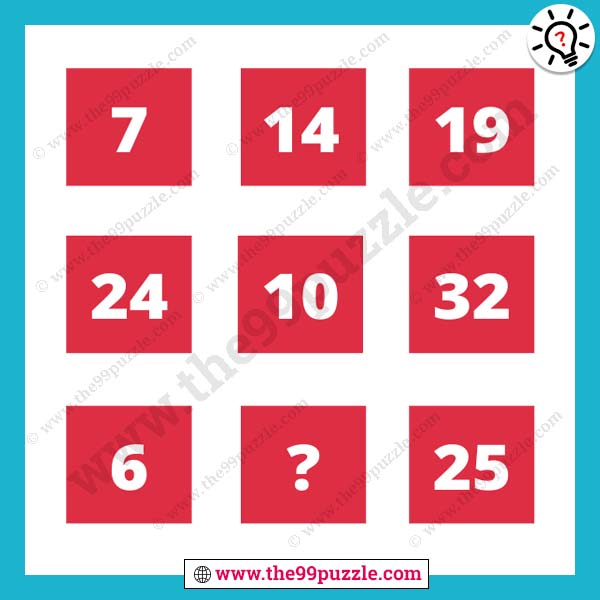# Easy mathematical puzzle for students with answer – Puzz273

This easy mathematical puzzle challenge for school students. How good are you at mathematical puzzles? Mathematical reasoning puzzles are asked in various examinations. Test your math skills to solve this type of puzzle. You learn maths shortcut tricks and math logic from this website. In this math logic puzzle, some numbers in the box. You have to crack the logic and replace the question mark.###### Explanation:

1st Row=19-7=12+2=14

2nd Row=32-24=8+2=10

3rd Row=25-6=19+2=21